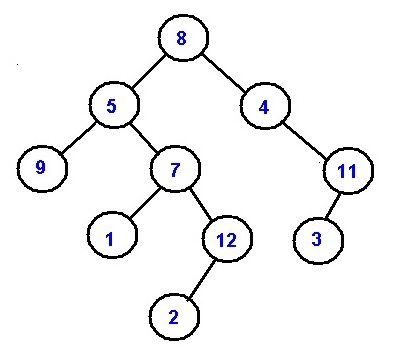Q:

# Ancestors in Binary Tree

Given a Binary Tree and a target key, write a function that prints all the ancestors of the key in the given binary tree.

Example:

Let's the tree be like following:```    Let for node value 12:
Ancestors are:
7, 5, 8
While for node value 7:
Ancestors are:
5, 8```

What is Ancestors?

For any node n,
Its ancestors are the nodes which are on the path between roots to node n

Thus for the above examples,

Example 1:

```    Node is 12 //represented by value
Root to the node path is
8->5->7->12
Thus the ancestors are 7, 5, 8
```

Example 2:

```    Node is 7 //represented by value
Root to the node path is
8->5->7
Thus the ancestors are 5, 8
```

Algorithm:

```FUNCTION printAncestors(Node *root, int target)
IF(!root)
return false;
IF( (root->left && root->left->data==target) ||
(root->right && root->right->data==target ) ||
printAncestors(root->left,target)||
printAncestors(root->right,target)){
Print root->data;
return true;
END IF
return false;
END FUNCTION
```

That simply means we are doing kind of DFS

For a currentnode to be ancestor of the target node the conditions are:

```1.  If the target node is its child node (either left child or right child)
//condition
IF((root->left && root->left->data==target) ||
(root->right && root->right->data==target ))

2.  If any of the two subtree of the current node contain ancestor of the target
node then the current node is also an ancestor.
//condition
IF(printAncestors(root->left, target)||
printAncestors(root->right, target))
```

Example with explanation:

```For target node 7:
Root 8 is ancestor on condition: its left subtree contains ancestor 5
5 is ancestor since target node is its right child
Thus ancestors are:
5, 8 //in order```

C++ Implementation:

``````#include <bits/stdc++.h>
using namespace std;

//tree node
class Node{
public:
int data;
Node *left;
Node *right;
};

bool printAncestors(Node *root, int target)
{
if(!root)
return false;

if(	(root->left && root->left->data==target) ||
(root->right && root->right->data==target ) ||
printAncestors(root->left,target)||
printAncestors(root->right,target)){
cout<<root->data<<" ";
return true;
}
return false;
}

//creating new nodes
Node* newnode(int data){
Node* node = (Node*)malloc(sizeof(Node));
node->data = data;
node->left = NULL;
node->right = NULL;
return(node);
}

int main() {
//**same tree is builted as shown in example**
cout<<"tree in the example is build here"<<endl;
//building the tree like as in the example
Node *root=newnode(8);
root->left= newnode(5);
root->right= newnode(4);
root->right->right=newnode(11);
root->right->right->left=newnode(3);
root->left->left=newnode(9);
root->left->right=newnode(7);
root->left->right->left=newnode(1);
root->left->right->right=newnode(12);
root->left->right->right->left=newnode(2);

int s;

cout<<"enter input value to find ancestors......"<<endl;
cin>>s;

printAncestors(root,s);
return 0;
}
``````

Output

```tree in the example is build here
enter input value to find ancestors......
7
5 8```